Wheel Direction of Rotation Detector
d. bodnar  revised 04-04-2015

Introduction
Recently a fellow garden railroader asked me if I could come up with a way for a passenger car to determine which way it was going so that appropriate lights could be illuminated.  The car is an observation car (from USA Trains, I believe) that is designed to illuminate exterior lights one way when it is moving forward and a different way when running backwards.

This is easily accomplished if the train operates from standard DC track power.  Unfortunately he was running either DCC or constant track power with radio control so that the polarity on the track always remains the same.  The same issue would arise when using battery power.

He had built a mechanism that threw a switch one way when running forward and the other way when going backwards but it put a good bit of drag on the car.  He was looking for a solution that produced little or no drag.  He had also considered a modification to the coupler so that a forward pull could be detected but that option was not practical with the couplers that he was using.

After giving this some thought (over a few days and a few bike rides!) I came up with a method using three magnets and a reed switch.  If the magnets are mounted as shown below the sequence of pulses that the reed switch produced was different based on direction of travel.

This diagram shows how the magnets are mounted on the wheel.  Three magnets are placed.  Two are 60 degrees apart and the third is 120 degrees away from the second, leaving a 180 degree gap between the third and first magnet.

When the wheel turns in a clockwise direction the pulses are short, long, medium, short, long medium.  When going counter clockwise they are short, medium, long, short, medium, long.  By determining which pulse is the long one and comparing that to the one that follows it the direction can be figured out.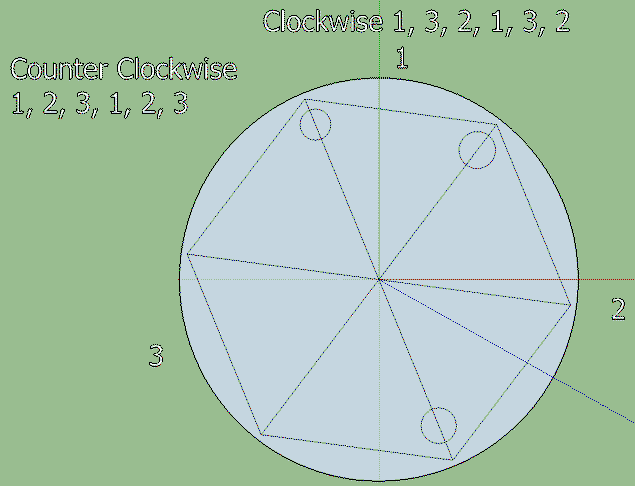Test Rig
This mechanism was used for testing.  The small motor (upper left) turns the wheel either clockwise or counter clockwise.  The DPDT switch (circled in black) determines the direction or rotation.  The magnets (two of the three are circled in red) pulse the reed switch (circled in yellow).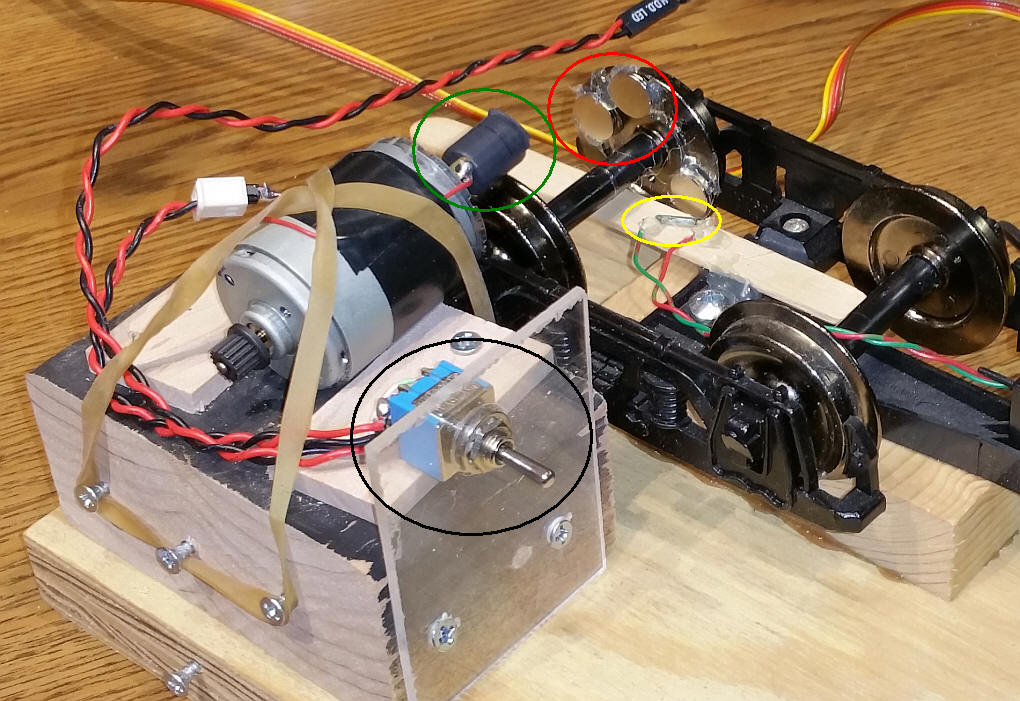Circuit
The reed switch is mounted next to the magnets on the wheel.  There is a duplicate switch on the prototype board that can be used for testing.  The yellow LED lights as the wheel turns and detects magnets.  One red LED lights if the wheel turns in one direction and the other red LED lights if it turns the other way.  After a 5 second period without any magnet detection passes both LEDs are turned off.

The diagram shows the circuit with an Arduino Uno but just about any Arduino should work.  This is especially important if space is an issue.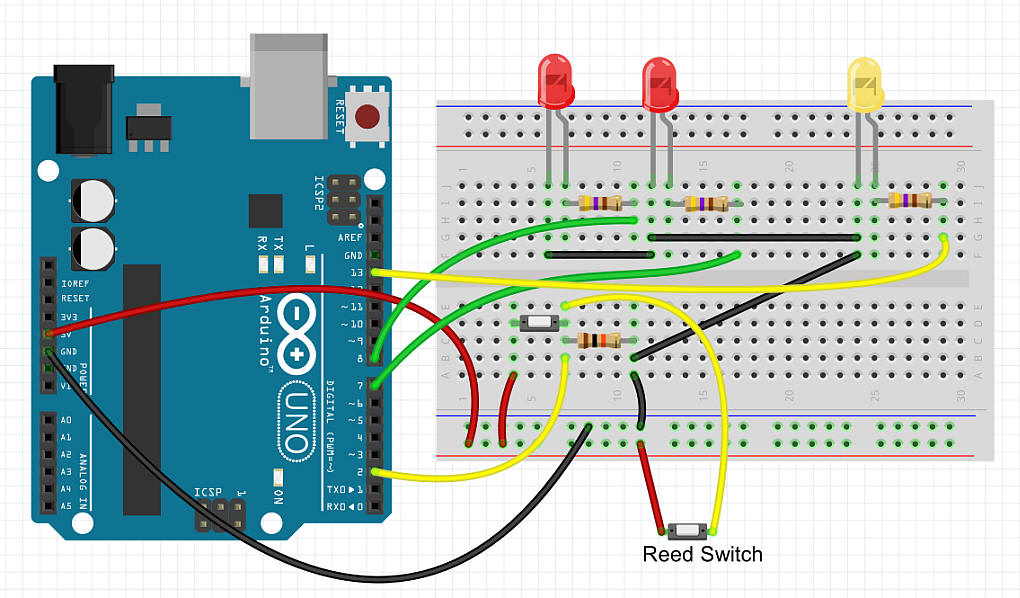Software -1
Initial testing used the example program that comes with the Arduino called "Button".  It simply blinks an LED when a button (or reed switch) closes.  I attached one wire from the reed switch to pin 2 on the Arduino.  A 10K resistor pulled that pin low by going to ground.  The other wire from the reed switch connected to + 5 volts.
 ```/* Button Turns on and off a light emitting diode(LED) connected to digital pin 13, when pressing a pushbutton attached to pin 2. The circuit: * LED attached from pin 13 to ground * pushbutton attached to pin 2 from +5V * 10K resistor attached to pin 2 from ground * Note: on most Arduinos there is already an LED on the board attached to pin 13. created 2005 by DojoDave modified 30 Aug 2011 by Tom Igoe This example code is in the public domain. http://www.arduino.cc/en/Tutorial/Button */ // constants won't change. They're used here to // set pin numbers: const int buttonPin = 2; // the number of the pushbutton pin const int ledPin = 13; // the number of the LED pin // variables will change: int buttonState = 0; // variable for reading the pushbutton status void setup() { // initialize the LED pin as an output: pinMode(ledPin, OUTPUT); // initialize the pushbutton pin as an input: pinMode(buttonPin, INPUT); } void loop(){ // read the state of the pushbutton value: buttonState = digitalRead(buttonPin); // check if the pushbutton is pressed. // if it is, the buttonState is HIGH: if (buttonState == HIGH) { // turn LED on: digitalWrite(ledPin, HIGH); } else { // turn LED off: digitalWrite(ledPin, LOW); } }```
Logic Analyzer Test
I connected my Saleae Logic Analyzer to the output LED on the Arduino and made the following observations.

Wheel clockwise rotation
Note that the large period (1) has a short one (3) to the right and a medium length one to the left (2).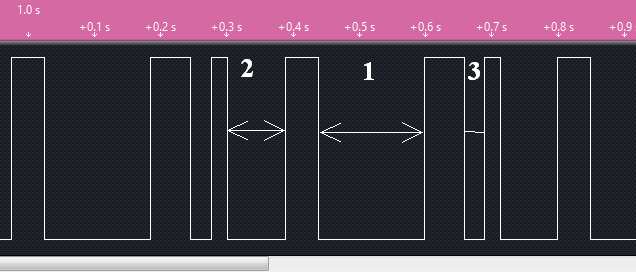Wheel counter clockwise rotation
Note that the large period (1) has a medium one (2) to the right and a short length one to the left (3).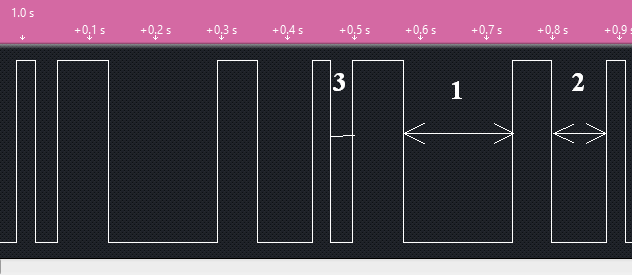Note that the two pulse patterns are surely different.  All that remains is to write a computer program for the Arduino to differentiate between them.

Software -2
The first program that I wrote measures the length of each pulse gap and sends it to the serial terminal:
 ```/* Sample sketch to show pulses from reed switch on wheel to determine if the wheel turns CW or CCW three magnets on wheel at 1/6ths - two together and one two away NOTE: this version gives a good result and definitely discriminates between forward and backward now to do the math and get it to report properly! */ const int buttonPin = 2; // the number of the pushbutton pin const int ledPin = 13; // the number of the LED pin // variables will change: int buttonState = 0; // variable for reading the pushbutton status int minusCount = 0; int plusCount = 0; int firstPlusFlag =0; int firstMinusFlag = 0; int countValue; int y=0; void setup() { Serial.begin(115200); // initialize the LED pin as an output: pinMode(ledPin, OUTPUT); // initialize the pushbutton pin as an input: pinMode(buttonPin, INPUT); Serial.println("forward / backward wheel test - 3-29-15"); } void loop(){ buttonState = digitalRead(buttonPin); if (buttonState == HIGH) { plusCount = plusCount + 1; firstMinusFlag=0; if (minusCount !=0) { Serial.print("cnt "); Serial.println(minusCount); countValue[y]=minusCount; y=y+1; } minusCount = 0; digitalWrite(ledPin, HIGH); } else { minusCount = minusCount + 1; plusCount = 0; digitalWrite(ledPin, LOW); } if (y==6){ int big=0; for(int y=0;y<6;y++){ Serial.print(countValue[y]); Serial.print(" "); if (countValue[y] >= big){ big = countValue[y]; } //// countValue[y]=0; } Serial.println(""); Serial.print("big = "); Serial.println(big); big=0; y=0; // } } } ```
 ```Working Code - lights Red LED based on direction of travel - the wheel has to turn a bit more than one revolution to detect the direction of travel. Version Wheel_CWorCCW_v_2_0_Working``` ```/* Sample sketch to show pulses from reed switch on wheel to determine if the wheel turns CW or CCW three magnets on wheel at 1/6ths - two together and one two away */ const int buttonPin = 2; // the number of the pushbutton pin const int ledPin = 13; // the number of the LED pin const int ledDirection = 8; const int ledDirection2 = 7; // variables will change: int buttonState = 0; // variable for reading the pushbutton status long minusCount = 0; int firstPlusFlag =0; int firstMinusFlag = 0; long countValue; long maxCountValuePosition=0; long maxCountValuePosition2=0; int direction =0; long big=0; int y=0; unsigned long time; void setup() { Serial.begin(9600); // initialize the LED pin as an output: pinMode(ledPin, OUTPUT); pinMode(ledDirection, OUTPUT); pinMode(ledDirection2, OUTPUT); // initialize the pushbutton pin as an input: pinMode(buttonPin, INPUT); Serial.println("forward / backward wheel test V1.4x - 3-30-15"); time = millis(); } void loop(){ if(millis()-time >= 5000){ // if 5 seconds passes without a movement clear both LEDs digitalWrite(ledDirection, LOW); digitalWrite(ledDirection2, LOW); } buttonState = digitalRead(buttonPin); if (buttonState == HIGH) { firstMinusFlag=0; if (minusCount !=0) { Serial.print("cnt "); Serial.print(y); Serial.print(" = "); Serial.print (minusCount); Serial.print(" "); countValue[y]=minusCount; if (countValue[y] >= big){ big = countValue[y]; maxCountValuePosition=y; } y=y+1; } minusCount = 0; digitalWrite(ledPin, HIGH); time=millis(); } else { minusCount = minusCount + 1; digitalWrite(ledPin, LOW); } if (y==3){ Serial.println(""); for (int x=0;x<3;x++){ Serial.print(x); Serial.print(" "); Serial.println(countValue[x]); } Serial.println(""); Serial.print("big = "); Serial.print(big); Serial.print(" pos = "); Serial.print(maxCountValuePosition); Serial.print(" "); big=0; y=0; countValue=countValue; direction = (countValue[maxCountValuePosition]/countValue[maxCountValuePosition+1]); Serial.print(" Ratio = "); Serial.println(direction); if (direction >=4 ){ digitalWrite(ledDirection, HIGH); digitalWrite(ledDirection2, LOW); } else{ digitalWrite(ledDirection, LOW); digitalWrite(ledDirection2, HIGH); } } } ```

O-Scale with Hall Sensor
The photo below shows a test rig for O-Scale.  There are three small magnets (circled in yellow) that stimulate a small Hall Effect Sensor (circled in red).

Two adjustments to the software are needed.  See notes after the photo.  The first change is necessary as the Hall sensor is high when no magnet is sensed and low when a magnet is detected.  The sensor's output goes to pin 2 and is pulled high with a 10K resistor.  The sensor's other pins go to +5 volts and ground.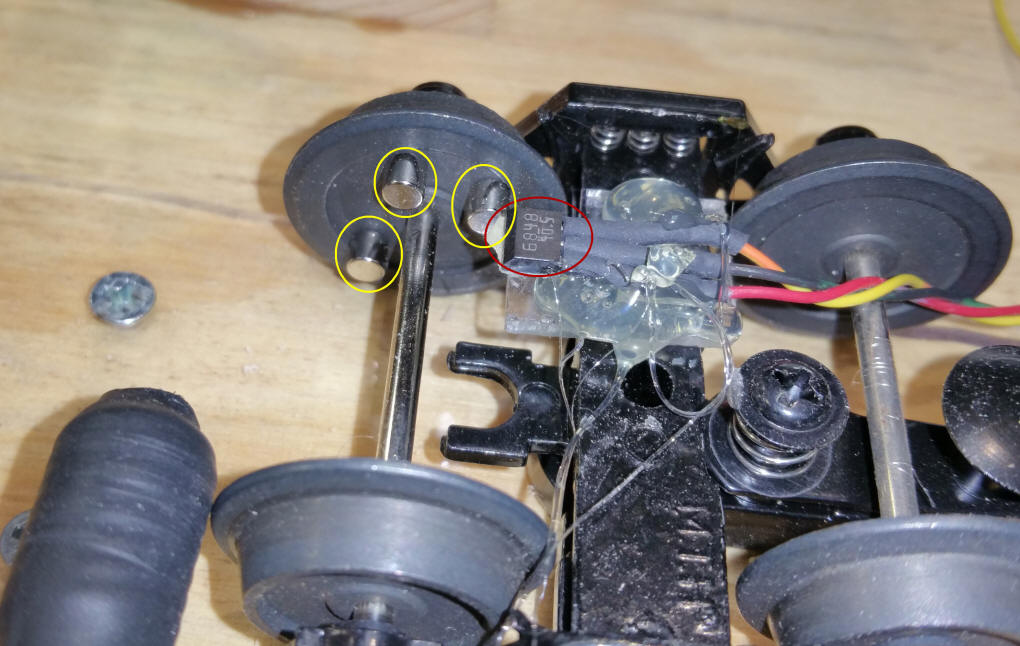`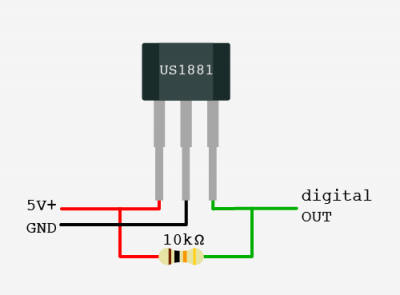`
``` buttonState = digitalRead(buttonPin);
if (buttonState == LOW) {     /////////////////////////Change here for HALL
firstMinusFlag=0;```
` `
``` Serial.print("  Ratio = ");
Serial.println(direction);
if (direction >=2 ){    /////////////////////////Change here for HALL
digitalWrite(ledDirection, HIGH);
digitalWrite(ledDirection2, LOW);```

PIC Schematic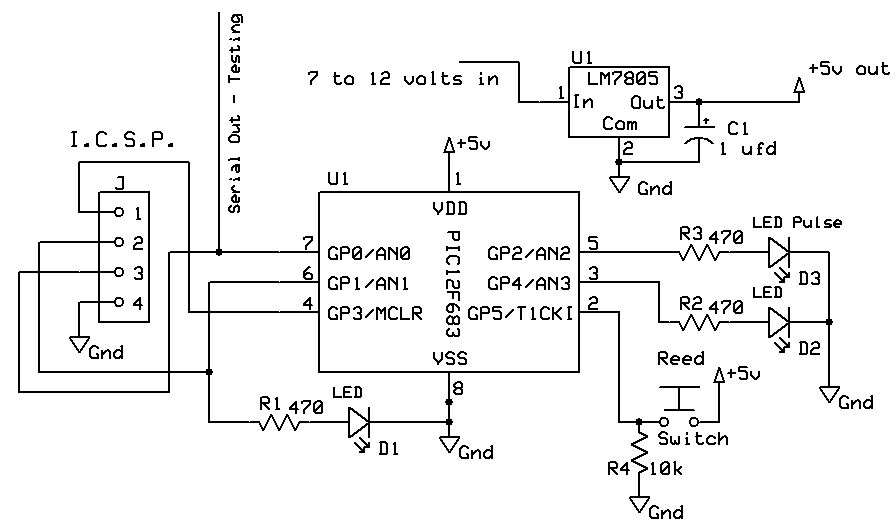PIC Basic Pro Code - version 1.0
I translated the Arduino code (written in "C") to Basic for the PIC 12F683 - The code is very similar to that of the Arduino
` `
``` ' d. bodnar 4-4-2015 Version 1.1
'Sample sketch to show pulses from reed switch on wheel to determine if the wheel turns CW or CCW

Include "modedefs.bas"
ansel = 0
cmcon0 = 7
Serial_out      var gpio.0  'pin 7 'Serial Out
LEDPulse        var gpio.2  'pin 5 hpwm 1 - Pulses when reed hit
LEDDirection1   var gpio.4  'pin 3 an3
LEDDirection2   var gpio.1  'pin 6 an1
ReedSW          var gpio.5  'pin 2 - reed switch
notUsed3        var gpio.3  'pin 4
Temp1           var word
Temp2           var word
b1              var byte
b2              var byte
'Serial does not work if next line is compiled
'''OSCCON = %00100000 ' Set to 8MHz
Serout serial_out,n9600,[13,10,13,10,13,10,"d. bodnar  Ver 1.1 + Wheel Direction",13,10]
Serout serial_out,n9600,[13,10,"8 MhZ 4-04-15",13,10]
gpio = %00100000        '5 is only input - others outputs

minusCount var word
firstPlusFlag var byte
firstMinusFlag var byte
countValue  var word 
maxCountValuePosition var word
maxCountValuePosition2 var word
direction var byte
big var word
y var byte
x var byte
SerPrintFlag var bit
SerPrintFlag=0
Top:

if reedSW=1 then
firstMinusFlag =0
if (minusCount <>0) then
countValue[y]=minusCount
if (countValue[y]> big) then
big = countValue[y]
maxCountValuePosition=y
endif
y=y+1
endif
minusCount=0
high ledpulse
else
minusCount=minusCount+1
low ledpulse
endif

if y=3 then
for x=0 to 3
if SerPrintFlag=1 then	  Serout serial_out,n9600,[ #countValue[x],"  "]
next x
if SerPrintFlag=1 then	  Serout serial_out,n9600,["  big= ", #big, " pos = ",#maxCountValuePosition," " ]
big =0
y=0
countValue=countValue
direction=countValue[maxCountValuePosition]/countValue[maxCountValuePosition+1 ]
if SerPrintFlag=1 then	Serout serial_out,n9600,["   Ratio = ",#direction ,13,10]
if direction >2 then
high leddirection1
low leddirection2
else
low leddirection1
high leddirection2
endif
endif

Goto Top:  ```
Two Hall Sensor Schematic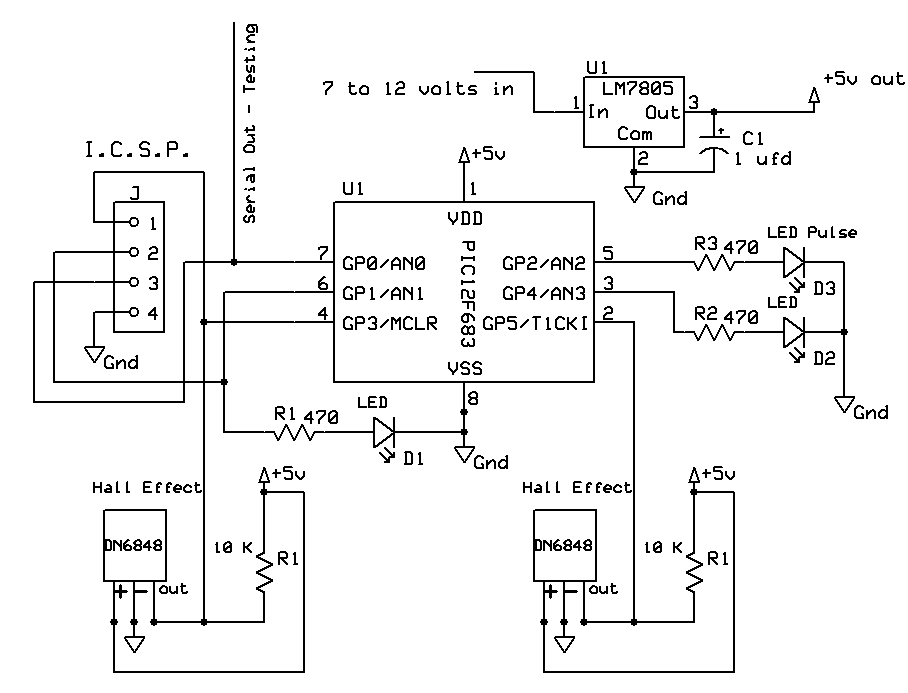`PIC BASIC PRO - uses two Hall Sensors & one magnet`
` `
``` ' d. bodnar 4-4-2015 Version 1.3 WORKING
'Sample sketch to show pulses from reed switch on wheel to determine if the wheel turns CW or CCW

Include "modedefs.bas"
ansel = 0
cmcon0 = 7
Serial_out      var gpio.0  'pin 7 'Serial Out
LEDPulse        var gpio.2  'pin 5 hpwm 1 - Pulses when reed hit
LEDDirection1   var gpio.4  'pin 3 an3
LEDDirection2   var gpio.1  'pin 6 an1
Hall1           var gpio.5  'pin 2 - reed switch
Hall2           var gpio.3  'pin 4

Serout serial_out,n9600,[13,10,13,10,13,10,"d. bodnar  Ver 1.3 + Wheel Direction",13,10]
Serout serial_out,n9600,[13,10,"8 MhZ 4-04-15",13,10]

gpio = %00101000        '5 and 3 are inputs - others outputs

FreeCount var word
low ledDirection1:low leddirection2
FreeCount=0

Top:
FreeCount=freecount + 1
if Hall1 = 0 and freecount > 5000 then
Freecount=0
high LEDDirection1
low LEDdirection2
endif
if Hall2 = 0 and freecount > 5000 then
freecount=0
high LEDDirection2
low LEDdirection1
endif

goto top:```